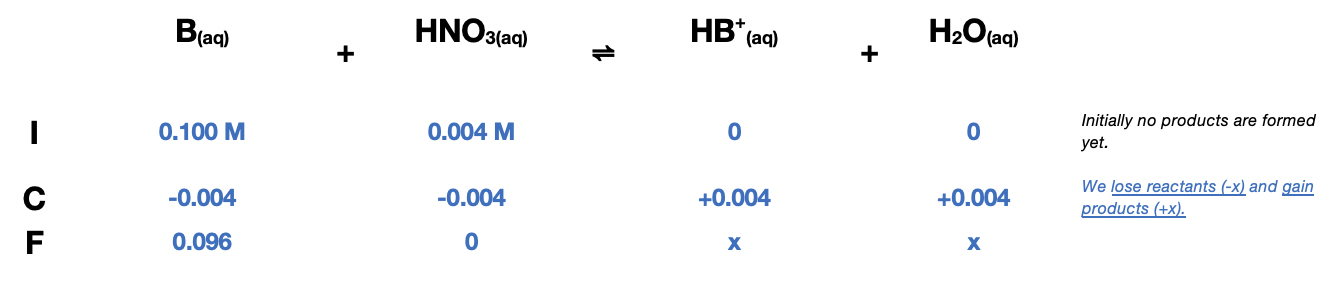# Problem: A student dissolves 0.0100 mole of an unknown weak base in 100.0 mL water and titrates the solution with 0.100 M HNO3. After 40.0 mL of 0.100 M HNO 3 was added, the pH of the resulting solution was 8.00. Calculate the Kb value for the weak base.

###### FREE Expert Solution

Recall:

$\overline{){{\mathbf{K}}}_{{\mathbf{b}}}{\mathbf{=}}\frac{\mathbf{products}}{\mathbf{reactants}}}$

We shall construct an ICF Chart first:

0.004 molesWe shall construct an ICE Chart based on the following reactions with water:

HB+ + H2O ⇌ B + H3O+

$\overline{){\mathbf{pOH}}{\mathbf{=}}{\mathbf{14}}{\mathbf{-}}{\mathbf{pH}}}$

${\mathbf{pOH}}{\mathbf{=}}{\mathbf{14}}{\mathbf{-}}{\mathbf{8}}{\mathbf{=}}$6.00

$\overline{)\mathbf{\left[}{\mathbf{OH}}^{\mathbf{-}}\mathbf{\right]}{\mathbf{=}}{{\mathbf{10}}}^{\mathbf{-}\mathbf{pOH}}}$

$\mathbf{\left[}{\mathbf{OH}}^{\mathbf{-}}\mathbf{\right]}{\mathbf{=}}{{\mathbf{10}}}^{\mathbf{-}\mathbf{6}}$

Calculate the concentrations:

Total volume = 100 mL WB + 40 mL HNO3 = 140 mL

0.0286 M

98% (355 ratings)###### Problem Details

A student dissolves 0.0100 mole of an unknown weak base in 100.0 mL water and titrates the solution with 0.100 M HNO3. After 40.0 mL of 0.100 M HNO 3 was added, the pH of the resulting solution was 8.00. Calculate the Kb value for the weak base.